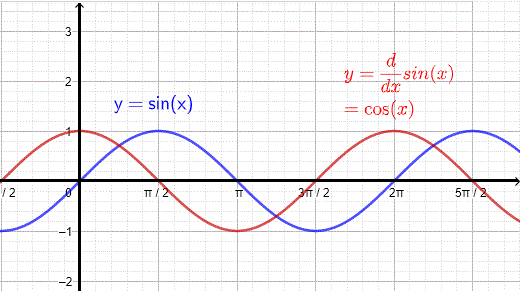# Proof of Derivative of sin x

The proof of the derivative of $\sin (x)$ is presented using the definition of the derivative. The derivative of a sine composite function is also presented including examples with their solutions.

## Proof of the Derivative of sin x Using the Definition

The definition of the derivative $f'$ of a function $f$ is given by
$f'(x) = \lim_{h \to 0} \dfrac{f(x+h)-f(x)}{h}$
Let $f(x) = \sin(x)$ and write the derivative of $\sin(x)$ as a limit
$f'(x) = \lim_{h \to 0} \dfrac{\sin(x+h)-\sin(x)}{h}$
Use the formula $\sin(x+h) = \sin(x)\cos(h) + \cos(x)\sin(h)$ to rewrite the derivative of $sin(x)$ as
$f'(x) = \lim_{h \to 0} \dfrac{\sin(x)\cos(h)+\cos(x)\sin(h)-\sin(x)}{h}$
Rewrite $f'(x)$ as follows
$f'(x) = \lim_{h \to 0} \dfrac{\sin(x) (cos(h) - 1) + \cos(x) \sin(h))}{h}$
Use the theorem: the limit of the sum of functions is equal to the sum of the limits of these functions to rewrite $f'(x)$ as follows
$f'(x) = \lim_{h \to 0} \dfrac{\sin(x) (cos(h) - 1)}{h} + \lim_{h \to 0} \dfrac{\cos(x) \sin(h)}{h}$
Rewrite the above as
$f'(x) = \sin(x) \lim_{h \to 0} \dfrac{ (cos(h) - 1)}{h} + \cos(x) \lim_{h \to 0} \dfrac{ \sin(h)}{h}$

We now use the following results on the limits of trigonometric functions
$\lim_{h \to 0} \dfrac{\sin(h)}{h} = 1$ , proved in the
use of squeezing theorem to find limits of mathematical functions .
$\lim_{h \to 0} \dfrac{cos(h) - 1}{h} = 0$ , proved in
calculate limits of trigonometric functions
to simplify $f'(x)$ to
$f'(x) = \sin(x) (0) + \cos(x) (1) = cos(x)$

conclusion
$\displaystyle {\dfrac {d}{dx} \sin x = \cos x }$

## Graph of sin x and its Derivative

The graphs of $\sin(x)$ and its derivative are shown below. Note that at any minimum or maximum of $\sin(x)$ corresponds a zero of the derivative $\cos(x)$. Also for any interval over which $\sin(x)$ is increasing the derivative is positive and for any interval over which $\sin(x)$ is decreasing, the derivative is negative.## Derivative of the Composite Function sin(u(x))

Let us consider the composite function sin of another function u(x). Use the chain rule of differentiation to write

$\displaystyle \dfrac{d}{dx} \sin (u(x)) = (\dfrac{d}{du} \sin u) (\dfrac{d}{dx} u )$

Simplify

$= \cos u \dfrac{d}{dx} u$

Conclusion

$\displaystyle \dfrac{d}{dx} \sin (u(x)) = \cos u \dfrac{d}{dx} u$

Example 1
Find the derivative of the composite sin functions

1. $f(x) = \sin (x^2-x)$
2. $g(x) = \sin (\sin(x))$
3. $h(x) = \sin \left(\dfrac{1-x}{1+x} \right)$

Solution to Example 1

1. Let $u(x) = x^2-x$ and therefore $\dfrac{d}{dx} u = \dfrac{d}{dx} (x^2-x) = 2x - 1$ and apply the rule for the composite sin function given above

$\displaystyle \dfrac{d}{dx} f(x) = \cos u \dfrac{d}{dx} u = \cos (x^2-x) \times (2x-1)$

$= (2x-1) \cos (x^2-x)$

2. Let $u(x) = \sin x$ and therefore $\dfrac{d}{dx} u = \dfrac{d}{dx} \sin x = \cos x$. Apply the above rule of differentiation for the composite sin function

$\displaystyle \dfrac{d}{dx} g(x) = \cos u \dfrac{d}{dx} u = \cos (\sin x) \times (\cos x)$

$= \cos x \cos (\sin x)$

3. Let $u(x) = \dfrac{1-x}{1+x}$ and therefore $\dfrac{d}{dx} u = -\dfrac{2}{(1+x)^2}$ and apply the rule of differentiation for the composite sin function obtained above

$\displaystyle \dfrac{d}{dx} h(x) = \cos u \dfrac{d}{dx} u = \cos (\dfrac{1-x}{1+x}) \times ( -\dfrac{2}{(1+x)^2})$

$= -\dfrac{2}{(1+x)^2} \cos (\dfrac{1-x}{1+x})$